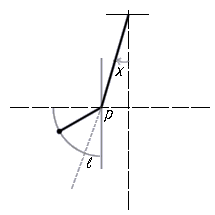### Constrained Pendulum

 A constrained pendulum example prepared for Eurosim can be viewed on their site - follow the links to Comparison 7. The problem was posed to present abilities to solve boundary value problems, handling of state events and analysis of linear approximation of the nonlinear model.mlx'' + dlx' + mgsin(x) = 0 Events -- arrive_pin:   x' => x'l/(l-p)                  depart_pin: x' => x'(l-p)/l This boundary value problem -- finding initial conditions producing a given deflection of the shortened pendulum -- illustrates the modeling of state events characterized by string encounters with a pin located in the pendulum path of motion. The state events (arrive_pin/depart_ pin) act as modifiers of the equations of motion parameters and states.